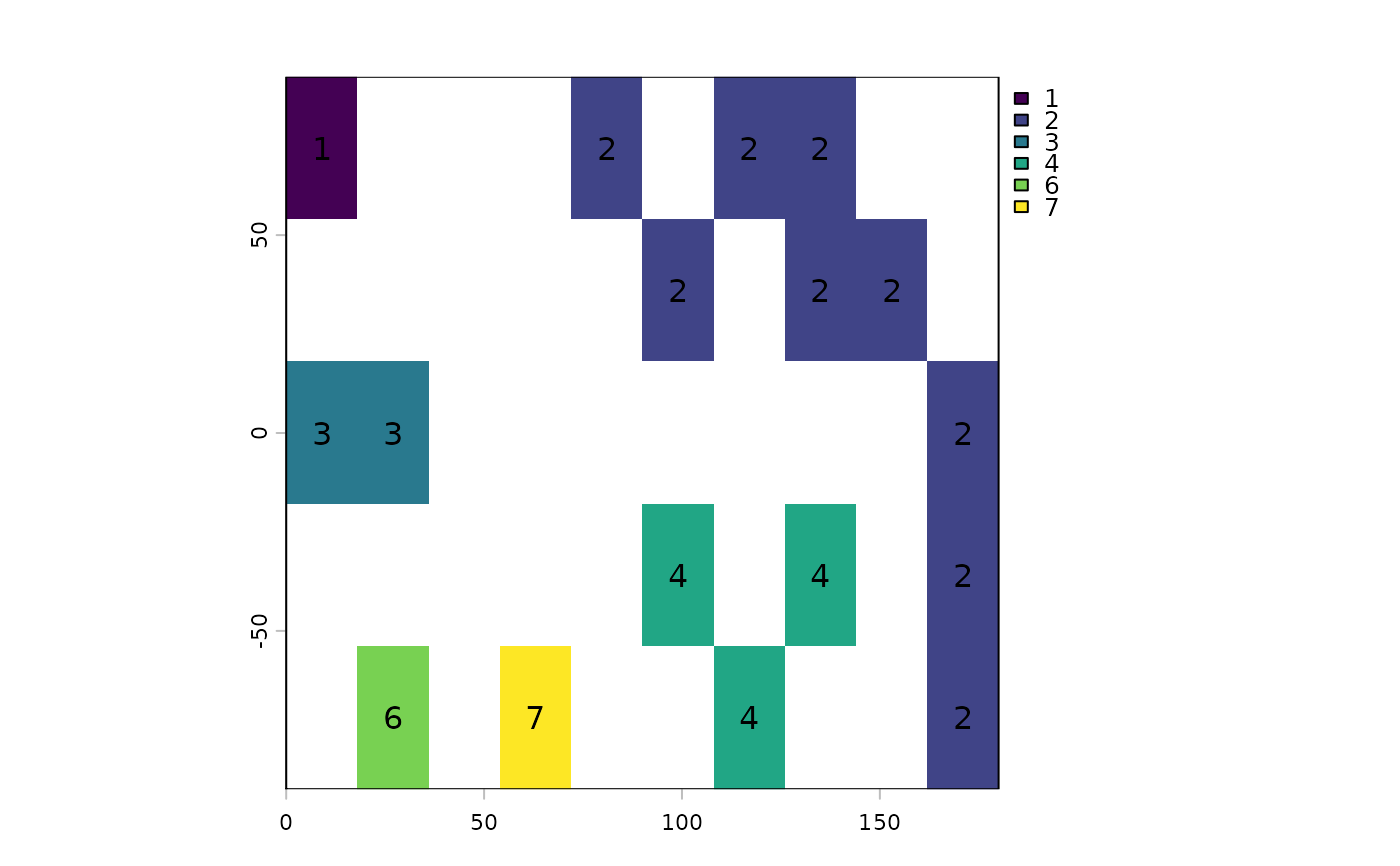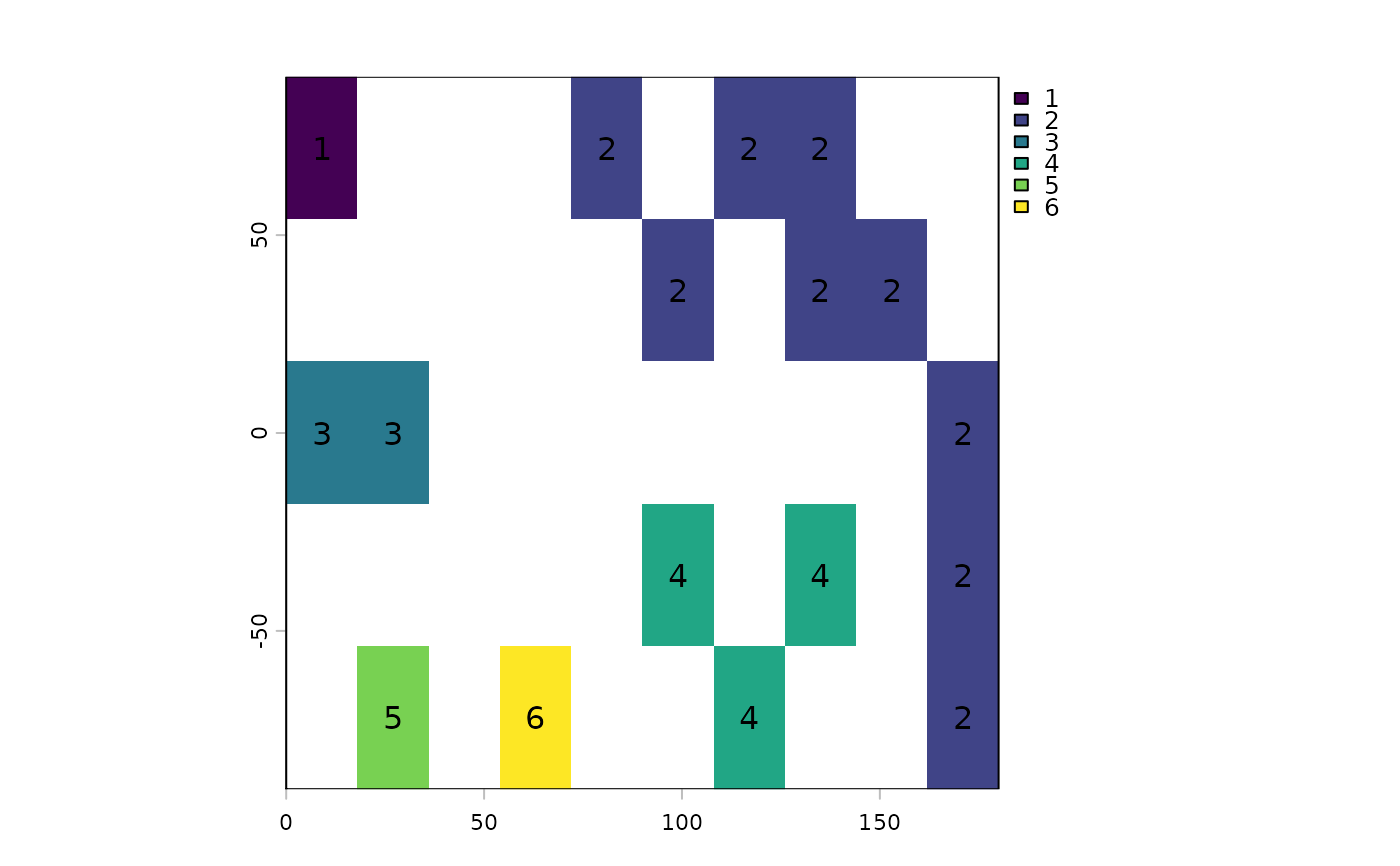Detect patches (clumps). Patches are groups of cells that are surrounded by cells that are NA. Set zeroAsNA to TRUE to also identify patches separated by cells with values of zero.

# S4 method for SpatRaster
patches(x, directions=4, zeroAsNA=FALSE, allowGaps=TRUE, filename="", ...)

## Arguments

x

SpatRaster

directions

integer indicating which cells are considered adjacent. Should be 8 (Queen's case) or 4 (Rook's case)

zeroAsNA

logical. If TRUE treat cells that are zero as if they were NA

allowGaps

logical. If TRUE there may be gaps in the patch IDs (e.g. you may have patch IDs 1, 2, 3 and 5, but not 4). If it is FALSE, these numbers will be recoded from 1 to the number of patches (4 in this example)

filename

character. Output filename

...

options for writing files as in writeRaster

## Value

SpatRaster. Cell values are patch numbers

focal, boundaries

## Examples

r <- rast(nrows=18, ncols=36, xmin=0)
r[1:2, 5:8] <- 1
r[5:8, 2:6] <- 1
r[7:12, 22:36] <- 1
r[15:16, 18:29] <- 1
p <- patches(r)

# zero as background instead of NA
r <- rast(nrows=10, ncols=10, xmin=0, vals=0)
r[3, 3] <- 10
r[4, 4] <- 10
r[5, 5:8] <- 12
r[6, 6:9] <- 12

# treat zeros as NA

p4 <- patches(r, zeroAsNA=TRUE)
p8 <- patches(r, 8, zeroAsNA=TRUE)

### patches for different values
# remove zeros manually
rr <- classify(r, cbind(0, NA))

# make layers for each value
s <- segregate(rr, keep=TRUE, other=NA)
p <- patches(s)

### patch ID values are not guaranteed to be consecutive
r <- rast(nrows=5, ncols=10, xmin=0)
set.seed(0)
values(r)<- round(runif(ncell(r))*0.7)
rp <- patches(r, directions=8, zeroAsNA=TRUE)
plot(rp, type="classes"); text(rp)## unless you set allowGaps=FALSE
rp <- patches(r, directions=8, zeroAsNA=TRUE, allowGaps=FALSE)
plot(rp, type="classes"); text(rp)### use zonal to remove small patches
f <- system.file("ex/elev.tif", package="terra")
r <- rast(f)
x <- classify(r, cbind(-Inf, 400, NA))
y <- patches(x)
# remove patches smaller than 100 ha
rz <- zonal(cellSize(y, unit="ha"), y, sum, as.raster=TRUE)
s <- ifel(rz < 250, NA, y)